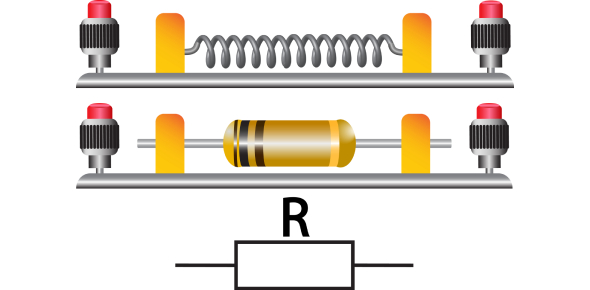# Quiz: Resistors MCQ By Gibilisco

20 Domande | Attempts: 834
ShareSettingsThis clearly illustrated introduction to electricity and electronics makes learning about them simple. This quiz on resistors contains MCQs by Gibilsico, who is a well-known writer. The quiz contains various questions ranging from easy, medium, to hard levels that not only gauge your knowledge of the subject but would also provide you with valuable information that will enhance your conceptual understanding.

• 1.
Proper biasing in an amplifier circuit
• A.

Causes it to oscillate

• B.

Prevents an impedance match

• C.

Can be obtained using a voltage divider network

• D.

Maximizes current flow

• 2.
A transistor can be protected from needless overheating by
• A.

A current-limiting resistor

• B.

Bleeder resistors

• C.

Maximizing the drive

• D.

Shorting out the power supply when the circuit is off

• 3.
A bleeder resistor
• A.

Is connected across the capacitor in a power supply

• B.

Keeps a transistor from drawing too much current

• C.

Prevents an amplifier from being overdriven

• D.

Optimizes the efficiency of an amplifier

• 4.
Carbon-composition resistors
• A.

Can handle gigantic levels of power

• B.

Have capacitance or inductance along with resistance

• C.

Have essentially no capacitance or inductance

• D.

Work better for ac than for dc

• 5.
A logical place for a wirewound resistor is
• A.

• B.

In a circuit where a noninductive resistor is called for

• C.

• D.

In a high-power dc circuit

• 6.
A metal-film resistor
• A.

Is made using a carbon-based paste

• B.

Does not have much inductance

• C.

Can dissipate large amounts of power

• D.

Has considerable inductance

• 7.
What type of resistor, or combination of resistors, would you use as the meter-sensitivity control in a test instrument, when continuous adjustment is desired?
• A.

A set of switchable, fixed resistors

• B.

A linear-taper potentiometer

• C.

An audio-taper potentiometer

• D.

A wirewound resistor

• 8.
What type of resistor, or combination of resistors, would you use as the volume control in astereo compact-disc (CD) player?
• A.

A set of switchable, fixed resistors

• B.

A linear-taper potentiometer

• C.

An audio-taper potentiometer

• D.

A wirewound resistor

• 9.
If a sound triples in actual power level, approximately what is this, expressed in decibels?
• A.

+3 dB

• B.

+5 dB

• C.

+6 dB

• D.

+9 dB

• 10.
Suppose a sound changes in volume by −13 dB. If the original sound power is 1.0 W, what is the final sound power?
• A.

13 W

• B.

77 mW

• C.

50 mW

• D.

There is not enough information given here to answer this question

• 11.
The sound from a portable radio is at a level of 50 dB. How many times the threshold ofhearing is this, in terms of actual sound power?
• A.

50

• B.

169

• C.

5000

• D.

100,000

• 12.
An advantage of a rheostat over a potentiometer is the fact that
• A.

A rheostat can handle higher frequencies

• B.

A rheostat is more precise

• C.

A rheostat can handle more current

• D.

A rheostat works better with dc

• 13.
A resistor is specified as having a value of 68 Ω, but is measured with an ohmmeter as 63 Ω.The value is off by which of the following percentages?
• A.

7.4%

• B.

7.9%

• C.

5%

• D.

10%

• 14.
Suppose a resistor is rated at 3.3 kΩ 5%. This means it can be expected to have a value between
• A.

2970 Ω and 3630 Ω

• B.

3295 Ω and 3305 Ω

• C.

3135 Ω and 3465 Ω

• D.

2.8 kΩ and 3.8 kΩ

• 15.
A package of resistors is rated at 56 Ω 10%. You test them with an ohmmeter. Which ofthe following values indicates a reject?
• A.

50.0 Ω

• B.

53.0 Ω

• C.

59.7 Ω

• D.

61.1 Ω

• 16.
A resistor has a value of 680 Ω, and you expect that it will have to draw 1 mA maximumcontinuous current in a circuit you’re building. What power rating is good for this application,but not needlessly high?
• A.

1⁄4 W

• B.

1⁄2 W

• C.

1 W

• D.

2 W

• 17.
Suppose a 1-kΩ resistor will dissipate 1.05 W, and you have a good supply of 1-W resistors of various ohmic values. If there’s room for 20 percent resistance error, the cheapest solution is to use
• A.

Four 1-kΩ, 1-W resistors in series-parallel

• B.

A pair of 2.2-kΩ, 1-W resistors in parallel

• C.

A set of three 3.3-kΩ, 1-W resistors in parallel

• D.

A single 1-kΩ, 1-W resistor, because all manufacturers allow for a 10 percent margin of safety when rating resistors for their power-handling capability

• 18.
Suppose a carbon-composition resistor has the following colored bands on it: red, red, red, gold. This indicates a resistance of
• A.

22 Ω

• B.

220 Ω

• C.

2.2 kΩ

• D.

22 kΩ

• 19.
The actual resistance of the component described in the previous question can be expected tovary above or below the specified ohmic value by up to what amount?
• A.

11 Ω

• B.

110 Ω

• C.

22 Ω

• D.

220 Ω

• 20.
Suppose a carbon-composition resistor has the following colored bands on it: gray, red, yellow. This unit can be expected to have a value within approximately what range?
• A.

660 kΩ to 980 kΩ

• B.

740 kΩ to 900 kΩ

• C.

7.4 kΩ to 9.0 kΩ

• D.

The manufacturer does not make any claim

## Related TopicsBack to top
×

Wait!
Here's an interesting quiz for you.